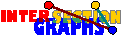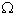# Duality

You may notice that there is some connection between this and this example. In the first we have the intersection graph of all attendance lists of the courses, whereas in the second we are considering the intersection graph of all course lists of the students. Both sets of lists obviously contain the same information. Each one is the dual of the other.

Duality is the most important, yet very natural, notion for intersection graphs. Assume there is a hypergraph H=(A,(Sx/xV)). For every aA, we define a* to be the set of those xV for which aSx.

The hypergraph (V,(a*/aA)) is called the dual hypergraph H* of H. Look at an example.

It should be clear that the dual hypergraph contains the same information as the original one, in fact it can easily be retrieved from the dual since H = (H*)*.

This essentially formal definition gets life by looking on intersection graphs. Let us consider the intersection graph G=(V,E) of the hypergraph H=(A,(Sx/xV)). Then the dual hyperedges a*, aA induce certain sugraphs in G, which we call star graphs in the following. These star graphs have two important features:

• Every star graph is complete, and
• The set of all star graphs forms an edge cover of G, i.e. every edge of G lies in at least one star graph.
We may think of the aA as detectors reporting all hyperedges covering it. Obviously two hyperedges of H intersect if and only if there is some detector reporting both. This is equivalent to the two statements above. Look at our example on how the star graphs of an intersection graph may look like.

Intersection graphs can also be defined by duality. The underlying graph (sometimes called 1-skeleton) of a hypergraph H=(A,(Sx/xV)) has the same vertex set A as H, and as edges all pairs of vertices that are covered by some hyperedge of H. Now(H) is just the underlying graph of H*---the star graphs are just the hyperedges of H*.

Note that in geometric intersection models there may be infinitely many star graphs, however only finitely many distinct. Moreoever, since most of these geometric models are stable under slight pertuberations, we may assume in most cases that every star graph occuring occurs infinitely often.Is there any connection between intersection graphs of dual hypergraphs? Given two graphs G1 and G2, can you construct a hypergraph H such that G1 =(H) and G2 =(H*)?

For an example take the special case of competition and resource graphs of a digraph---they are intersection graphs of the family of all out-neighborhoods respectively in-neighborhoods, which are obviously dual to each other.

Duality is used for some natural approach for the recognition problem.

Now you may be interested in the special role of cliques in intersection graphs or go back to the start page for intersection graphs.

Erich Prisner